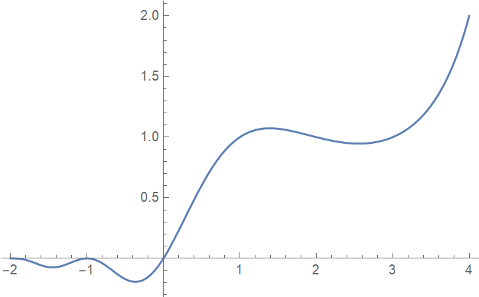# Superfactorial

The factorial of a positive integer n is the product of the numbers from 1 up to and including n:

n! = 1 × 2 × 3 × … × n.

The superfactorial of n is the product of the factorials of the numbers from 1 up to and including n:

S(n) = 1! × 2! × 3! × … × n!.

For example,

S(5) = 1! 2! 3! 4! 5! = 1 × 2 × 6 × 24 × 120 = 34560.

Here are three examples of where superfactorial pops up.

## Vandermonde determinant

If V is the n by n matrix whose ij entry is ij-1 then its determinant is S(n-1). For instance,V is an example of a Vandermonde matrix.

## Latin squares

The number of Latin squares of size n is bounded below by S(n). More on Latin squares and upper and lower bounds on how many there are here.

## Permutation tensor

One way to define the permutation symbol uses superfactorial:## Barnes G-function

The Barnes G-function extends superfactorial to the complex plane analogously to how the gamma function extends factorial. For positive integers n,Here’s plot of G(x)produced by

    Plot[BarnesG[x], {x, -2, 4}]

in Mathematica.